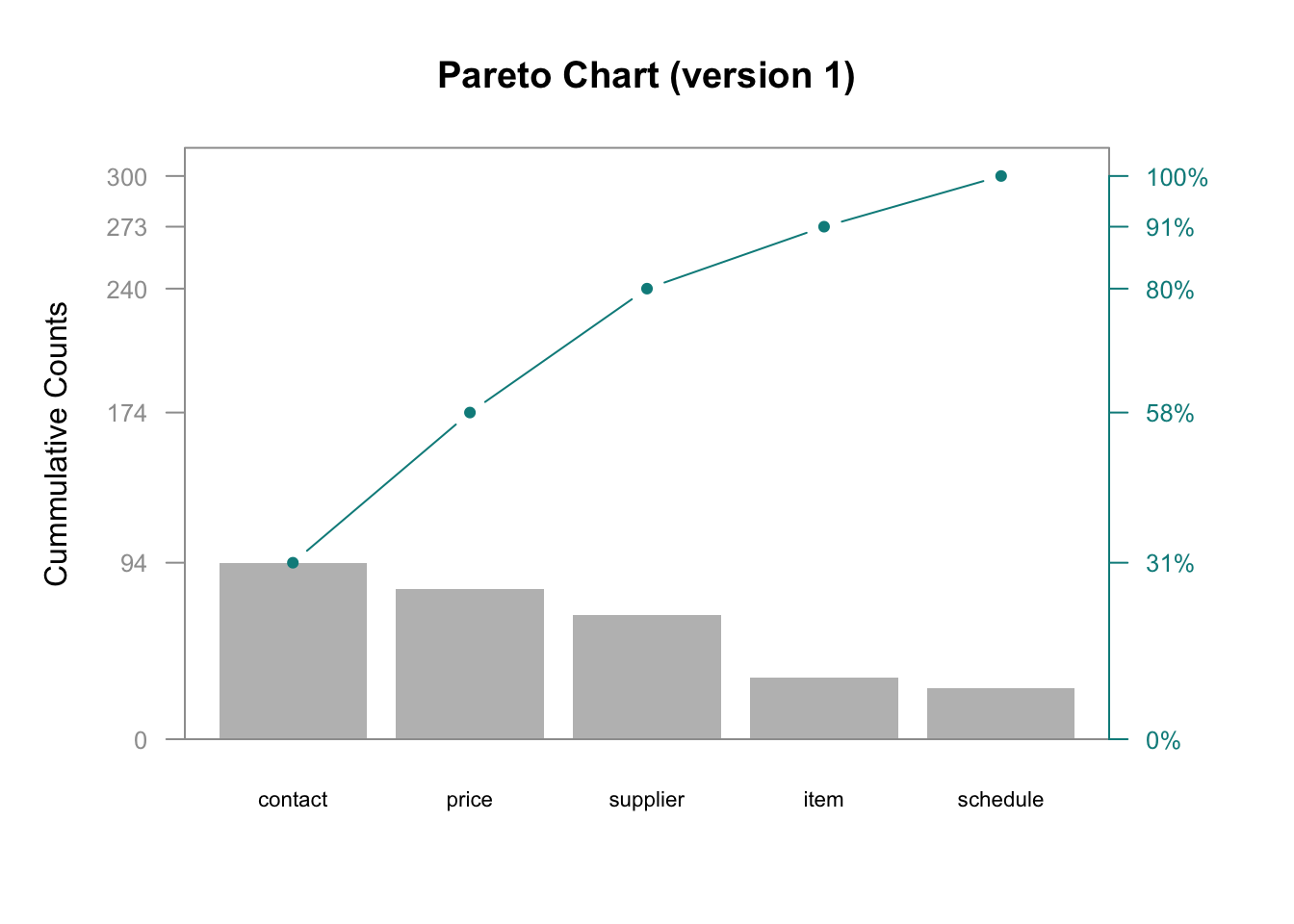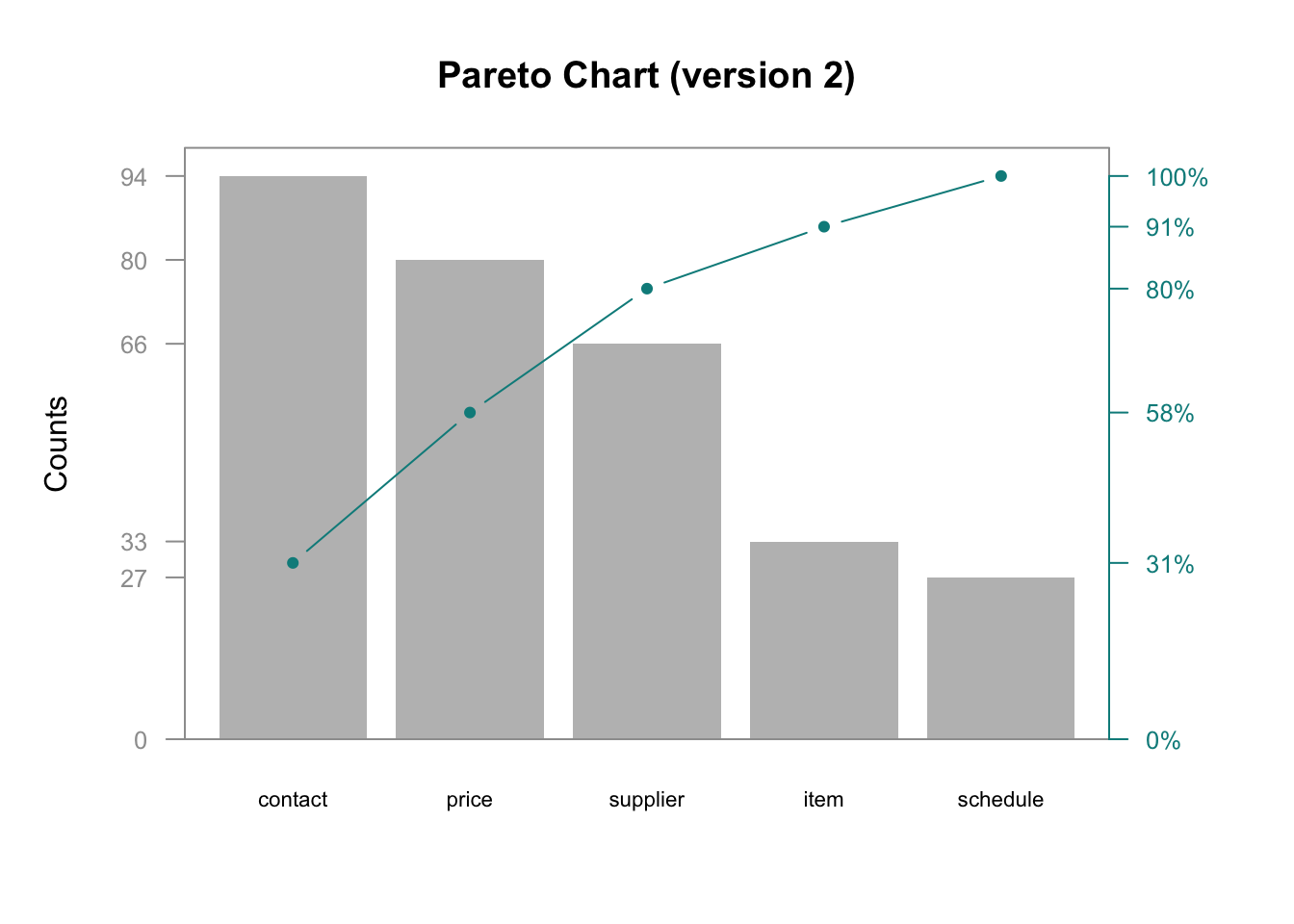Pareto chart, named after Vilfredo Pareto, is a type of chart that contains both bars and a line graph, where individual values are represented in descending order by bars, and the cumulative total is represented by the line. This type of chart is usefull in many ways and is in general a better idea to use Pareto Chart instead of pie chart since it reveals more information.

### Data Preparation

Assume we have some defect counts for different sources.

##   category defect
## 1    price     80
## 2 schedule     27
## 3 supplier     66
## 4  contact     94
## 5     item     33

To prepare data for Pareto Chart we need to sort counts in decreasing order, calculate cumulative sum and cumulative frequency of the counts. This can be done in variety of ways in R. In the example below preparation is done with dplyr R package.

## Data preparation
suppressPackageStartupMessages(library(dplyr))

d <- arrange(d, desc(defect)) %>%
mutate(
cumsum = cumsum(defect),
freq = round(defect / sum(defect), 3),
cum_freq = cumsum(freq)
)
d
##   category defect cumsum  freq cum_freq
## 1  contact     94     94 0.313    0.313
## 2    price     80    174 0.267    0.580
## 3 supplier     66    240 0.220    0.800
## 4     item     33    273 0.110    0.910
## 5 schedule     27    300 0.090    1.000

## Pareto Chart: Version 1## R code to generate Pareto Chart (version 1)

## Saving Parameters
def_par <- par()

## New margins
par(mar=c(5,5,4,5))

## bar plot, pc will hold x values for bars
pc = barplot(d$defect, width = 1, space = 0.2, border = NA, axes = F, ylim = c(0, 1.05 * max(d$cumsum, na.rm = T)),
ylab = "Cummulative Counts" , cex.names = 0.7,
names.arg = d$category, main = "Pareto Chart (version 1)") ## Cumulative counts line lines(pc, d$cumsum, type = "b", cex = 0.7, pch = 19, col="cyan4")

## Framing plot
box(col = "grey62")

axis(side = 2, at = c(0, d$cumsum), las = 1, col.axis = "grey62", col = "grey62", cex.axis = 0.8) axis(side = 4, at = c(0, d$cumsum), labels = paste(c(0, round(d$cum_freq * 100)) ,"%",sep=""), las = 1, col.axis = "cyan4", col = "cyan4", cex.axis = 0.8) ## restoring default paramenter par(def_par)  ## Pareto Chart: Version 2## ## R code to generate Pareto Chart (version 2) ## Saving Parameters def_par <- par() # New margins par(mar=c(5,5,4,5)) ## plot bars, pc will hold x values for bars pc = barplot(d$defect,
ylim = c(0, 1.05 * max(d$defect, na.rm = T)), ylab = "Counts" , cex.names = 0.7, names.arg = d$category,
axis(side = 2, at = c(0, d$defect), las = 1, col.axis = "grey62", col = "grey62", tick = T, cex.axis = 0.8) ## frame plot box( col = "grey62") ## Cumulative Frequency Lines px <- d$cum_freq * max(d$defect, na.rm = T) lines(pc, px, type = "b", cex = 0.7, pch = 19, col="cyan4") ## Annotate Right Axis axis(side = 4, at = c(0, px), labels = paste(c(0, round(d$cum_freq * 100)) ,"%",sep=""),
par(def_par)